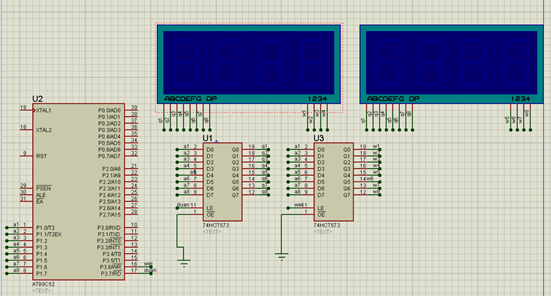01

2021

# 原理``````#include<reg52.h>
#include <intrins.h>
char code table[]={0xc0,0xf9,0xa4,0xb0,0x99,0x92,0x82,0xf8,0x80,0x90};

sbit duan=P3^7;
sbit wei=P3^6;

void delay(int t)
{
int x,y;

for(x=t;x>0;x--)
for(y=10;y>0;y--);
}
void nop()
{
_nop_();
_nop_();
}

//这几个变量是全局变量，主要用在主函数中
//不会影响到disp函数中的几个变量
int  abc,time,num,sec,min,hour;

/*************************************************
*该函数的功能作用是在开发箱的数码管显示时分秒
*开发箱上面的数码管从左往右是第0位到第7位
*在选择第几位的时候就给P1赋值2的第几位次方
*比如，显示在数码管的第3位，就给P1赋值2的3次方也就是8这个数值
***********************************************32***/
void disp(int sec, int min, int hour)
{

duan=0;
P1=table[hour/10];//显示时间的十位
duan=1;
duan=0;

wei=0;
P1=1;//显示在数码管的第0位。2的0次方等于1
wei=1;
wei=0;
delay(10);

duan=0;
P1=table[hour%10];//显示时间的个位
duan=1;
duan=0;

wei=0;
P1=2;//显示在数码管的第1位。2的1次方等于2
wei=1;
wei=0;
delay(10);

P1=table[min/10];//显示分钟的十位
duan=1;
duan=0;

P1=4;//显示在数码管的第2位。2的2次方等于4
wei=1;
wei=0;
delay(10);

P1=table[min%10];//显示分钟的个位
duan=1;
duan=0;

P1=8;//显示在数码管的第3位。2的3次方等于8
wei=1;
wei=0;
delay(10);

P1=table[sec/10];//显示秒钟的十位
duan=1;
duan=0;

P1=16;//显示在数码管的第4位。2的4次方等于16
wei=1;
wei=0;
delay(10);

P1=table[sec%10];//显示秒钟的个位
duan=1;
duan=0;

P1=32;//显示在数码管的第5位。2的5次方等于32
wei=1;
wei=0;
delay(10);
}//100

int year,month,date;

void disp2()
{
duan=0;
P1=table[year/1000];//显示年的千位
duan=1;
duan=0;

wei=0;
P1=1;//显示在数码管的第0位。2的0次方等于1
wei=1;
wei=0;
delay(10);

duan=0;
P1=table[year%1000/100];//百位
duan=1;
duan=0;

wei=0;
P1=2;//显示在数码管的第1位。2的1次方等于2
wei=1;
wei=0;
delay(10);

P1=table[year%100/10];//十位
duan=1;
duan=0;

P1=4;//显示在数码管的第2位。2的2次方等于4
wei=1;
wei=0;
delay(10);

P1=table[year%10];//个位
duan=1;
duan=0;

P1=8;//显示在数码管的第3位。2的3次方等于8
wei=1;
wei=0;
delay(10);

P1=table[month/10];//显示月的十位
duan=1;
duan=0;

P1=16;//显示在数码管的第4位。2的4次方等于16
wei=1;
wei=0;
delay(10);

P1=table[month%10];//显示月的个位
duan=1;
duan=0;

P1=32;//显示在数码管的第5位。2的5次方等于32
wei=1;
wei=0;
delay(10);

P1=table[date/10];//显示日期的十位
duan=1;
duan=0;

P1=64;//显示在数码管的第6位。2的6次方等于64
wei=1;
wei=0;
delay(10);

P1=table[date%10];//显示日期的个位
duan=1;
duan=0;

P1=128;//显示在数码管的第7位。2的7次方等于128
wei=1;
wei=0;
delay(10);

}
int show_flag = 0,show_time = 0;

void main (void)
{
//

/*定时器0初始化，定时时间50毫秒*/
TMOD=0x01;//定时器方式寄存器
TR0=1;//T0定时器/计数器的运行控制位
ET0=1;//中断允许寄存器中T0定时器/计数器的允许
TH0=(65536-50000)/256;
TL0=(65536-50000)%256;

EA=1;//中断控制的    总允许
/*********/

//时间初始化
year = 2021;
month = 5;
date = 24;
hour = 10;
min = 30;
sec = 50;

while(1)
{
if(sec==60)
{
sec=0;
min++;
if(min==60)
{
min=0;
hour++;

if(hour==24)
{
hour=0;
date++;
if(（month==4 || month==6 || month == 9 || month == 11） && date==31)
{
month++;
date = 1;
}
else if(month == 2)
{
//判断年是否为闰年，以此来判断date是28+1进1还是29+1进一

}
else if(month==1 || month==3 || month == 5 || month == 7|| month == 8 || month == 10 || month == 12） && date==32)
{
month++;
date = 1;
}

}
}
}

//每隔两秒切换一次显示
if(show_flag == 0)
disp(sec,min,hour);//显示时分秒
else
disp2();//显示年月日

}
}
//定时器0的中断服务函数，不需要主动调用
void time0() interrupt 1
{

TH0=(65536-50000)/256;
TL0=(65536-50000)%256;
time++;
//定时一次50毫秒，20次后是1秒，秒数+1
if(time==20)
{

time=0;
show_time++;
sec++;
//每隔两秒改变show_flag的值，如果要修改切换的时间，修改下面的2为其他整数就好
if(show_time%2 ==0)
{
show_flag = !show_flag;
}
}

}

``````

TAG